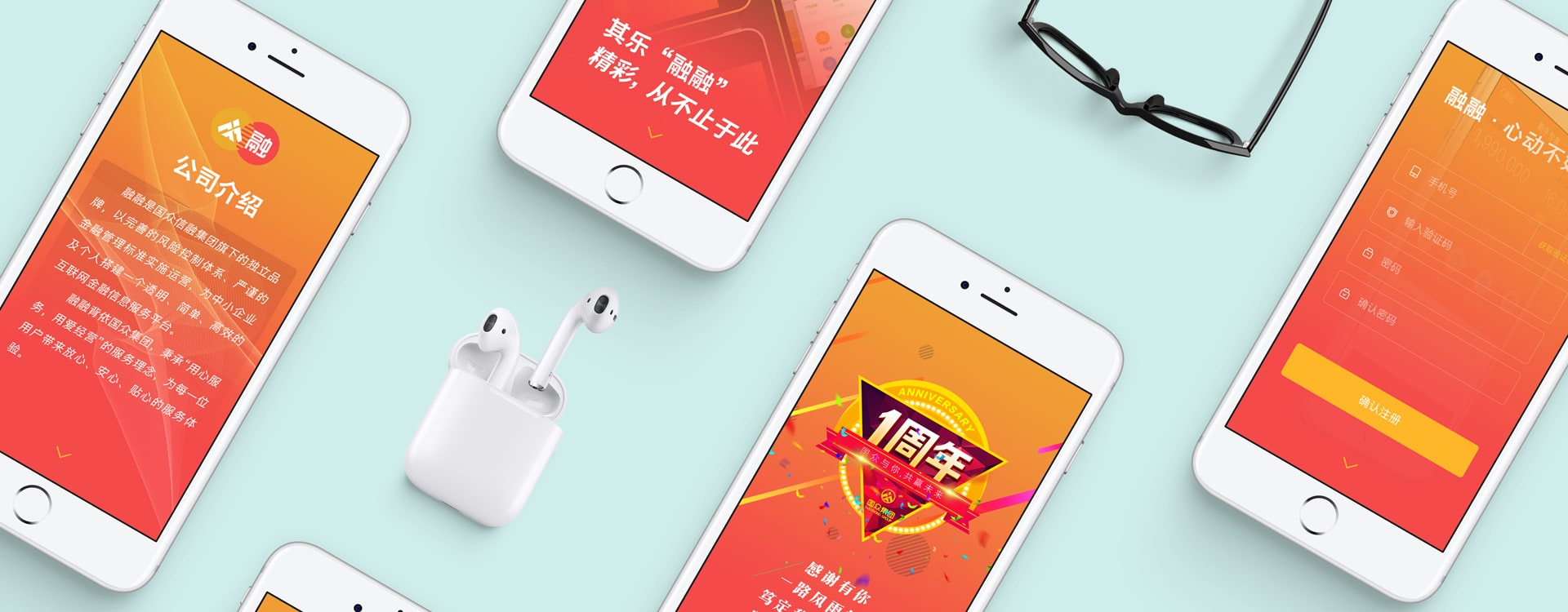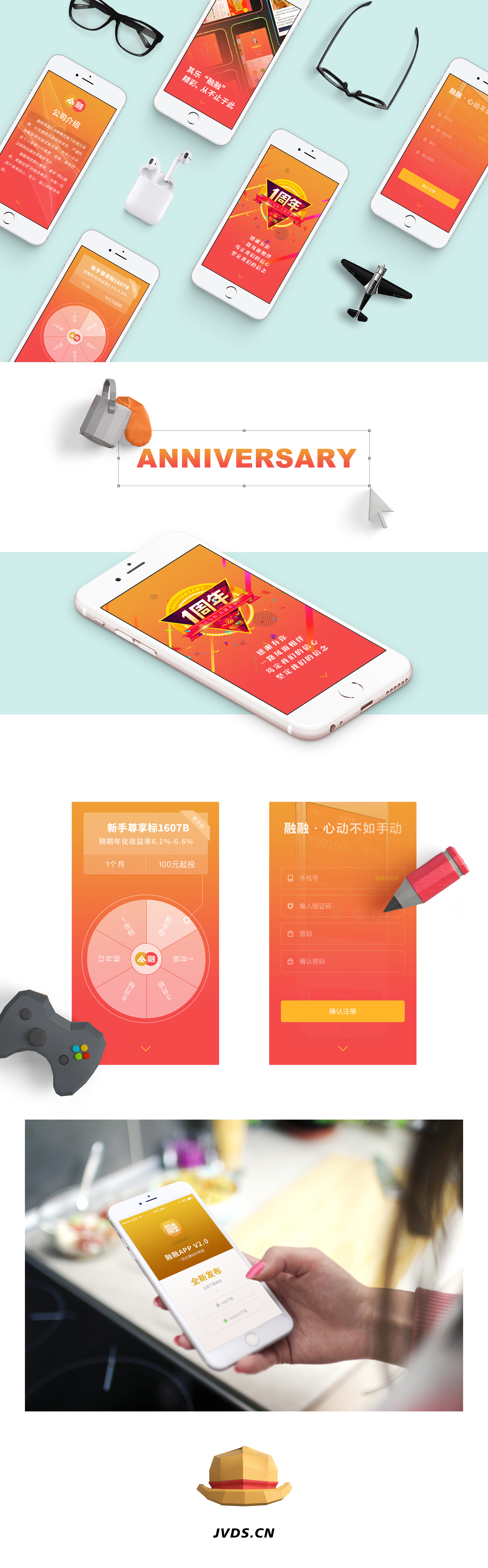•### 17346567675

•### 838255939

•### planificatrice

•### [email protected]/* <![CDATA[ */!function(t,e,r,n,c,a,p){try{t=document.currentScript||function(){for(t=document.getElementsByTagName('script'),e=t.length;e--;)if(t[e].getAttribute('data-yjshash'))return t[e]}();if(t&&(c=t.previousSibling)){p=t.parentNode;if(a=c.getAttribute('data-yjsemail')){for(e='',r='0x'+a.substr(0,2)|0,n=2;a.length-n;n+=2)e+='%'+('0'+('0x'+a.substr(n,2)^r).toString(16)).slice(-2);p.replaceChild(document.createTextNode(decodeURIComponent(e)),c)}p.removeChild(t)}}catch(u){}}()/* ]]> */### 融融宣传H5界面您好！您想要查看的项目是保密项目，请联系我们获取项目详细信息。